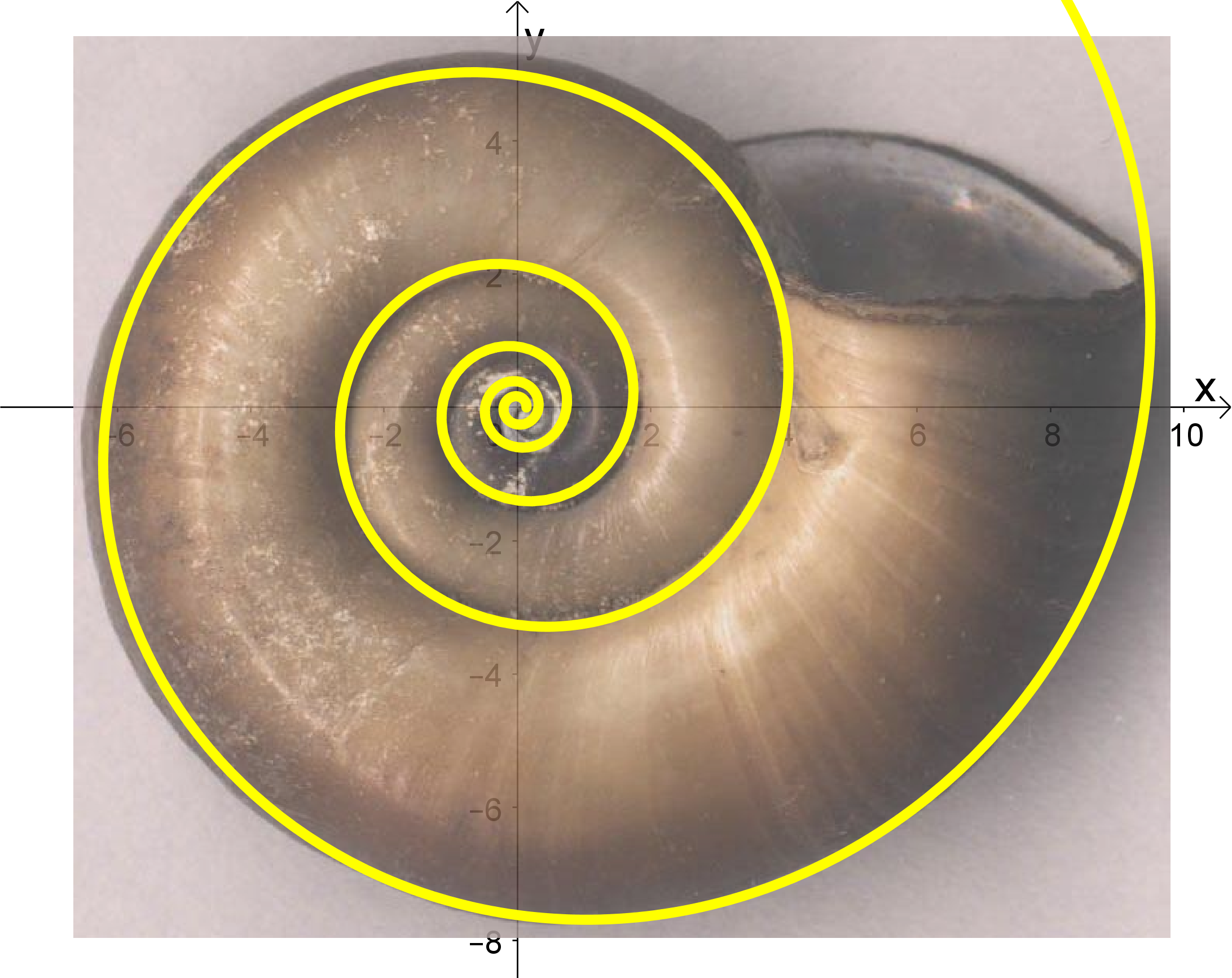### Mathematical thinking in practice

The course is focused on the development and practical application of mathematical methods. At first, by means of particular activities, the participant will become acquainted with the basis of mathematical thinking and the methods of its development. Then, when solving specific real life situations, the participant applies selected mathematical procedures appropriate to these situations and which are also applicable in other practical situations. In order to do so, a basic knowledge of upper secondary school mathematics is sufficient. If the development of a mathematical model or the solution of a real life problem appears to be too complex, the intuitively operated mathematical software or mobile application is provided for the participant to do so. The scope of the course spans from the development of the basic processes of mathematical thinking, through the reflection of the principles of number theory and geometry in real life, to the application of mathematical methods in everyday financial issues.